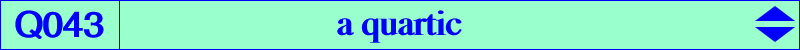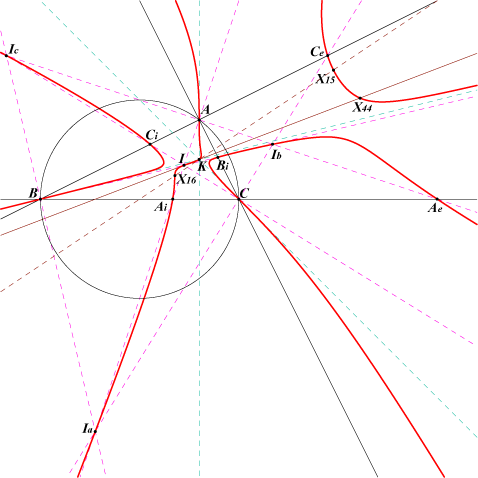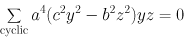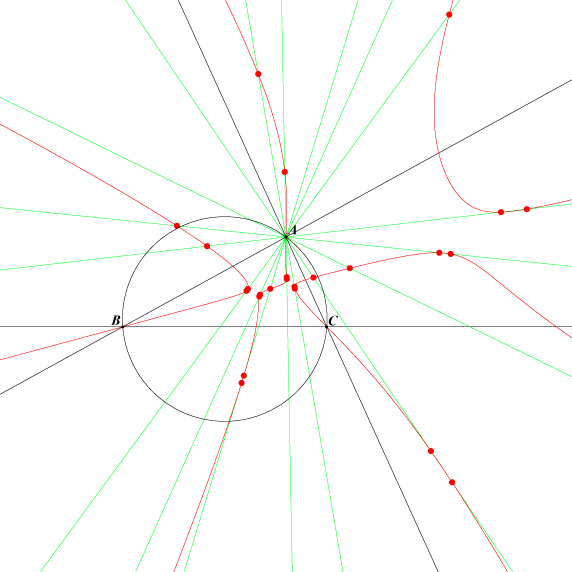X(1), X(6), X(15), X(16), X(44), X(3273), X(3274), X(3275) Ia, Ib, Ic : excenters extraversions of X(44) 6 feet of bisectors (Edward Brisse) 27 points with barycentrics : a sin((A+i 2pi)/3) : b sin((B+j 2pi)/3) : c sin((C+k 2pi)/3)] with i, j, k in {-1, 0, 1} among them X(3273), X(3274), X(3275). See below. six equilateral circumanticevian points (see Table 40)Q043 is the isogonal transform of Q042. It is analogous to Q002 and Q083. A construction is given in the page Q002. It is the locus of point P such that • P, its isogonal conjugate P* and G/P* are collinear. G/P* is the center of the circum-conic with perspector P* i.e. the G-Ceva conjugate of P*. • equivalently, X(6), P and P*/P are collinear. See Q002, locus property 9, for other similar curves. A, B, C are flexes with tangents the symmedians. Q043 is tangent at K to the Brocard axis. The tangents at the in/excenters pass through K.Q043 and the "X(358) sinus points" These 27 points lie on three groups of nine lines passing through the points A, B, C. They are analogous to the isogonal conjugates of the perspectors of ABC and the 27 Morley triangles since the Cosinus are replaced by Sinus in their coordinates.